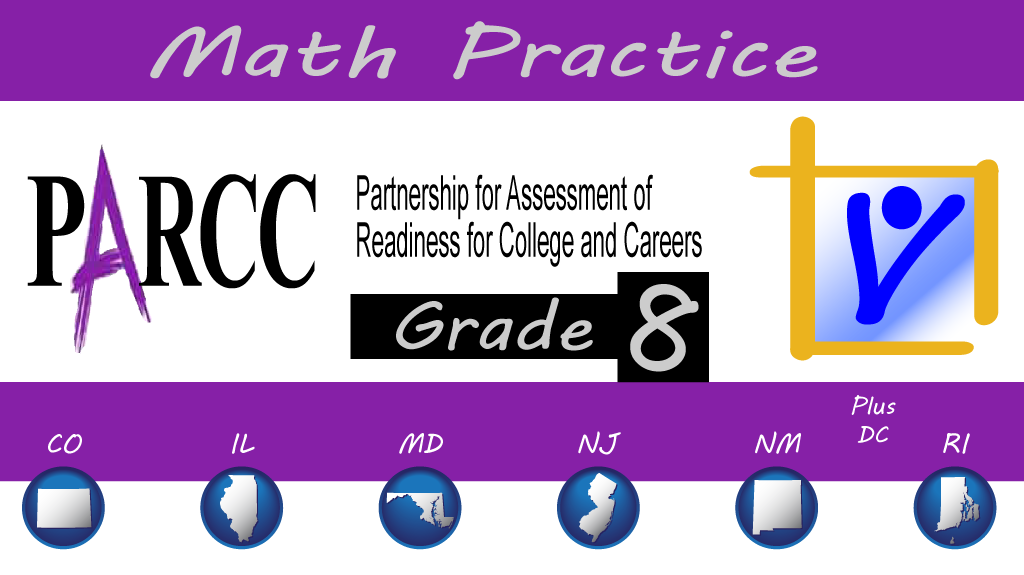Wednesday, September 30, 2020# Grade 8 PARCC math: integer exponents

#### The following multiple-select question, explained here in hopes of helping eighth-grade students and their parents in Maryland and Illinois prepare for the PARCC test near the end of this school year, appears on the released version of PARCC’s Spring 2016 test for grade eight math:Which expression is equivalent to$(7^3)^5 \cdotp 7^4$ ?

• A.$7^{3 \cdotp 5 \cdotp 4}$
• B.$7^{3 \cdotp 5 + 4}$
• C.$7^{3+5+4}$
• D.$7^{3(5+4)}$
• E.$7^{3 \cdotp 5} \textrm{ }\cdotp 7^4$
• F.$7^{3 + 5} \textrm{ }\cdotp 7^4$

Common Core Math Content 8th grade, Expressions and Equations, Work with radicals and integer exponents:

(8.EE.A.1) Know and apply the properties of integer exponents to generate equivalent numerical expressions. For example,$3^2 \times 3^{-5} = 3^{-3} = (\frac{1}{3})^{3} = \frac{1}{27}$

Solution strategy (there are others)

Apply the rules for exponents to each of the answer choices, since the base is the same in all cases: 7.

Any number a raised to a power mn equals$a^{m \cdotp n}$

In other words,$(7^3)^5 = 7^{3 \cdotp 5}$

And any number a raised to a power m + n equals$a^m \cdotp a^n$

In other words,$a^{m+n} = a^m \cdotp a^n \textrm{ or } 7^{15} \cdotp 7^4 = 7^{15+4}$

That means that any expression that equals 719 will be a match.

Consider A:$7^{3 \cdotp 5 \cdotp 4} = 7^{60} \textrm{ which is incorrect.}$

Consider B:$7^{3 \cdotp 5 + 4} = 7^{19} \textrm{ BINGO.}$

Consider C:$7^{3+5+4} = 7^{12} \textrm{ nope.}$

Consider D:$7^{3(5+4)} = 7^{3 \cdotp 9} = 7^{27} \textrm{ not happening.}$

Consider E:$7^{3 \cdotp 5} \textrm{ }\cdotp 7^4 = 7^{15} \cdotp 7^4 = 7^{15+4} \textrm{ works.}$

Consider F:$7^{3 + 5} \textrm{ }\cdotp 7^4 = 7^8 \cdotp 7^4 = 7^{12} \textrm{ not even close.}$

## Analysis of this question and online accessibility

Integer exponents with numerical bases are new for eighth graders under the Common Core. Students should know how to work with positive and negative exponents of a numerical base (fraction or integer), as well as with exponents that are themselves expressions.

If you or your parents need some practice, check out this worksheet with answers for the power rule and this one with more complete coverage of all the rules for exponents.

Multiple-choice questions like this can be delivered easily online and on paper-based tests, which makes this question accessible for students on any device they may use or on paper. Validity, reliability, and fairness measures should not differ significantly among the various delivery modes.

No special accommodation challenges can be identified with this question, so the question is considered fair.Paul Katula is the executive editor of the Voxitatis Research Foundation, which publishes this blog. For more information, see the About page.

### Busy pictures hinder reading comprehension

When extraneous illustrations are used less, children can have an easier time focusing on text and better reading comprehension.

### Student news roundup, Illinois, Sept. 28

Remote learning woes, BLM vs. ALM, inclusion and diversity, Halloween and fall, yearbook art, and a drive-in fall play.

### Ideas on teaching ceramics remotely

Teaching ceramics during a pandemic that includes all-remote learning is a little different, and some art teachers rise to the challenge.

### On constitutional flat taxes in Illinois

An important ballot question in IL involves the elimination of the flat tax in favor of a graduated income tax structure.

### Weather conference for Howard Co. 6th graders

The Howard County (Md.) Conservancy invites 6th graders to register for a conference about preparing for extreme weather.

### Exercise harder, remember more

Scientists have found that the more vigorously you exercise, the stronger the response in the brain that helps your memory.

### More than Covid keeps kids home at E. Peoria

Mud & debris flooded E Peoria Comm HS this summer, so students can't return to in-person learning sooner than the end of Oct.

### 1/3 of an Okla. school returns to quarantine

After only a few days of in-person instruction, an Okla. high school experienced a rise in Covid cases and has resumed remote learning.

### Schools rethink the whole idea of snow days

Why have snow days anymore if we can have 'virtual learning' days, now that we know a thing or two about how they work?

### Student news roundup, Maryland, Sept. 24

State to allow sports beginning in Oct., but some districts won't go back yet; Miss Maryland Agriculture; music lessons virtually.

### Grand jury indicts officer in Breonna Taylor case

A former police officer was indicted in connection with the death of Breonna Taylor in Louisville. But it was less than many had hoped for.